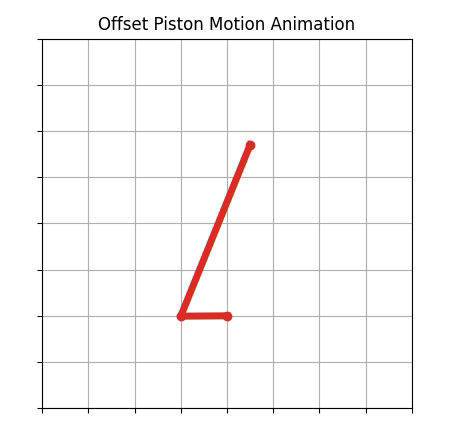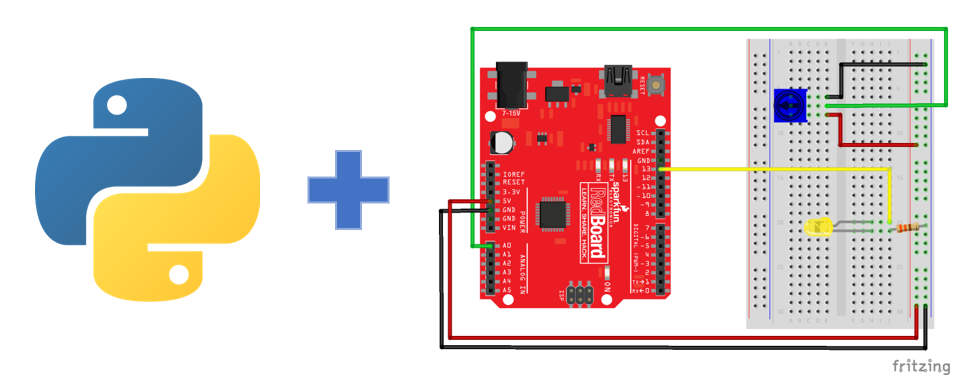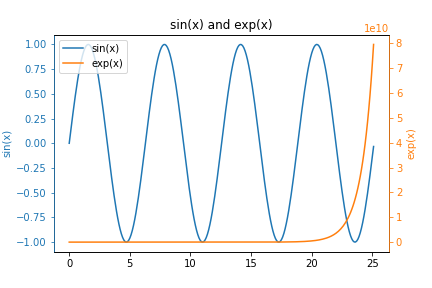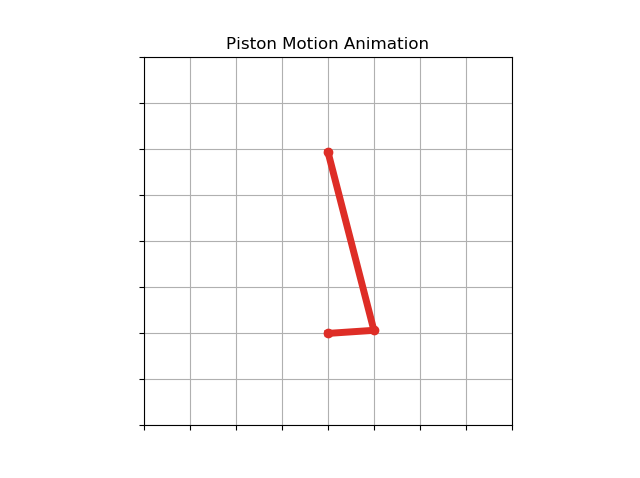## How to make animated plots with Matplotlib and PythonPython and Matplotlib can be used to create static 2D plots. But it Matplotlib can also be used to create dynamic auto-updating animated plots. In this post, you learn how to create a live auto-updating animated plot using Python and Matplotlib.

## Offset Piston Motion with Python and MatplotlibOffset piston motion is one of the classic types of engineering dynamics motion that belong to a category of 4-bar motion. Piston motion is the type of motion that the piston in a cylinder of a car engine goes through as the crankshaft rotates.

Offset piston motion has the same …

## Using Python and an Arduino to Read a SensorIn this post, you will see how to use Python to communicate with an Arduino and read a sensor. In particular, the sensor we'll use is a potentiometer. The potentiometer will connect to an Arduino microcontroller and the Arduino will communicate with a Python script using the PySerial package. Python …

## How to make y-y plots with MatplotlibIn this post, you will learn how to create y-y plots with Python and Matplotlib. y-y plots are a type of line plot where one line corresponds to one y-axis and another line on the same plot corresponds to a different y-axis. y-y plots typically have one vertical y-axis on …

## Matplotlib Animation to Demonstrate Piston MotionPiston motion is one of the classic types of motion in engineering dynamics that belong to a category of 4-bar motion. Piston motion is the type of motion that the piston in a cylinder of a car engine goes through as the engine's crankshaft rotates. In this post, we'll review …